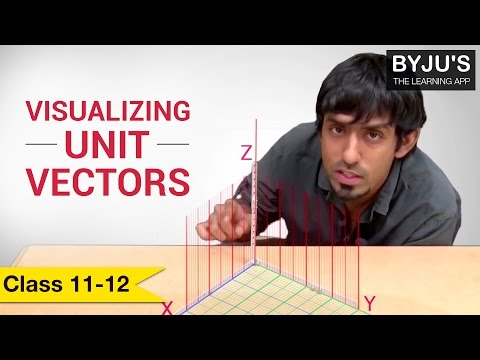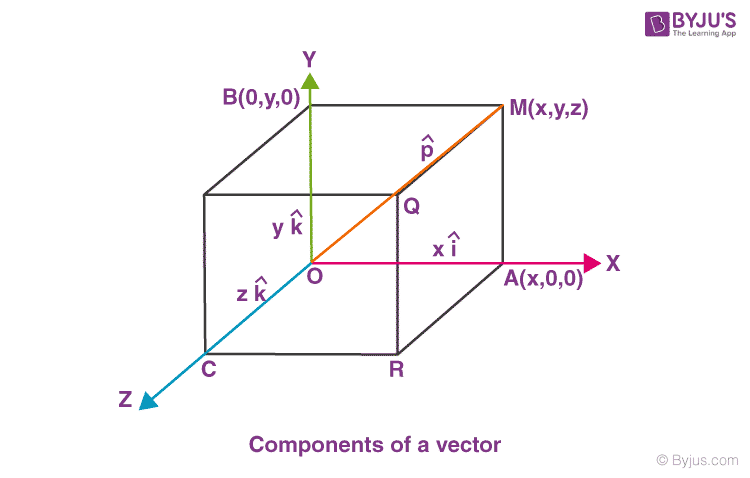# Visualizing Unit Vectors

The physical quantities for which both magnitude and direction are defined distinctly, are known as vector quantities. Vectors are denoted by putting an arrow over the denotations representing them. For example, to define the acceleration of a vehicle, along with its magnitude, its direction must also be specified. It can be represented in vector form as $$\vec{a}$$ m/s2. Vectors can be easily represented using the coordinate system in three dimensions. In this article, we will be discussing a concept known as the unit vector.

## What Is Unit Vector?

A unit vector is a vector that has a magnitude of 1 unit. A unit vector is also known as a direction vector. It is represented using a lowercase letter with a cap (‘^’) symbol along with it. A vector can be represented in space using unit vectors. Any vector can become a unit vector by dividing it by the vector’s magnitude as follows:

 $$unit\;vector=\frac{vector}{magnitude\;of\;the\;vector}$$

A unit vector p ̂having the same direction as vector $$\vec{p}$$ is given as:

 $$\hat{p}=\vec{p}|\vec{p}|$$

Where,
$$\hat{p}$$ represents a unit vector
$$\vec{p}$$ represents the vector
$$\vec{\left | p \right |}$$ represents the magnitude of the vector

It must be kept in mind that any two unit vectors $$\hat{p}$$ and $$\hat{q}$$ must not be considered as equal unit vectors just because they have the same magnitude. Since the direction in which the vectors are taken might be different therefore these unit vectors are different from each other. Therefore, to define a vector both magnitude and direction should be specified.## Component Form of Vector

In the Cartesian coordinate system, any vector $$\vec{p}$$ can be represented in terms of its unit vectors. The unit vectors in direction of x,y and z-axes are given by $$\hat{i}$$, $$\hat{j}$$ and $$\hat{k}$$ respectively. The position of vector $$\vec{p}$$ can be represented in space with respect to the origin of the given coordinate system as:

 $$\vec{p}$$ = x$$\hat{i}$$ + y$$\hat{j}$$ + z$$\hat{k}$$

The vector $$\vec{p}$$ can be resolved along the three axes as shown in the given figure. With OM as the diagonal, a parallel piped is constructed whose edges OA, OB and OC lie along the three perpendicular axes.From the above figure,

 $$\vec{OA}$$ = x$$\hat{i}$$ $$\vec{OB}$$ = y$$\hat{j}$$ $$\vec{OC}$$ = z$$\hat{k}$$

The vector $$\vec{p}$$ can be represented as

 $$\vec{p}=\vec{OM}=x\hat{i}+y\hat{j}+z\hat{k}$$

This is known as the component form of a vector. This represents the position of given vectors in terms of the three co-ordinate axes.

### What is a polar vector?

A polar vector is defined as the vector that has either a starting point or a application point. Velocity is an example of polar vector.

### What is the magnitude of a unit vector?

The magnitude of a unit vector is unity. Unit vector has only direction and no units or dimensions.

### What is the magnitude of a zero vector?

A zero vector is a null vector with zero magnitude. The best example of zero vector would be velocity of a stationary object.

### When can one say that the two vectors are equal?

Two vectors are said to be equal when they have the same magnitude and are parallel to each other.

### What happens to the direction when a vector is multiplied by a positive number?

The direction of the vector remains unchanged when a positive number is multiplied.

Test your Knowledge on unit vectors